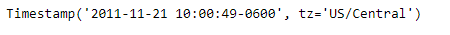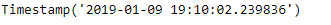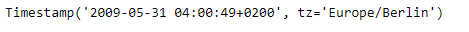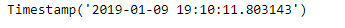Skip to content
Related Articles
Python | Pandas Timestamp.now
• Last Updated : 14 Jan, 2019

Python is a great language for doing data analysis, primarily because of the fantastic ecosystem of data-centric python packages. Pandas is one of those packages and makes importing and analyzing data much easier.

Pandas` Timestamp.now()` function return the current time in the local timezone. It is Equivalent to datetime.now([tz]).

Syntax :Timestamp.now()

Parameters : None

Return : Timestamp

Example #1: Use `Timestamp.now()` function to return the current time in the local timezone.

 `# importing pandas as pd``import` `pandas as pd`` ` `# Create the Timestamp object``ts ``=` `pd.Timestamp(year ``=` `2011``,  month ``=` `11``, day ``=` `21``,``           ``hour ``=` `10``, second ``=` `49``, tz ``=` `'US/Central'``)`` ` `# Print the Timestamp object``print``(ts)`

Output :Now we will use the `Timestamp.now()` function to find the current time in the local timezone.

 `# return the current time``ts.now()`

Output :As we can see in the output, the `Timestamp.now()` function has returned the current time in the local timezone. It auto detects the local timezone.

Example #2: Use `Timestamp.now()` function to return the current time in the local timezone.

 `# importing pandas as pd``import` `pandas as pd`` ` `# Create the Timestamp object``ts ``=` `pd.Timestamp(year ``=` `2009``, month ``=` `5``, day ``=` `31``,``       ``hour ``=` `4``, second ``=` `49``, tz ``=` `'Europe/Berlin'``)`` ` `# Print the Timestamp object``print``(ts)`

Output :Now we will use the `Timestamp.now()` function to find the current time in the local timezone.

 `# return the current time``ts.now()`

Output :As we can see in the output, the `Timestamp.now()` function has returned the current time in the local timezone. It auto detects the local timezone.

Attention geek! Strengthen your foundations with the Python Programming Foundation Course and learn the basics.

To begin with, your interview preparations Enhance your Data Structures concepts with the Python DS Course. And to begin with your Machine Learning Journey, join the Machine Learning – Basic Level Course

My Personal Notes arrow_drop_up